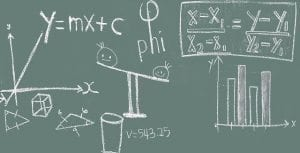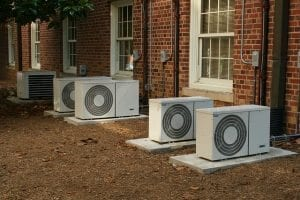# Your Guide to HVAC Formulas

Numbers play a big part in the HVAC industry. We don’t just mean the difference between a comfortable indoor 68 degrees and a too-cool 45 or muggy 95. No, an HVAC technician has to be familiar with a lot of other numbers and calculations to help make those comfortable conditions possible.

There’s a lot of tools you can use to get the right numbers, like checking reference books or using an app. But it’s also important to understand how they work.

Knowing some of the basic formulas involved in HVAC work will help you work faster and more reliably. While there’s nothing wrong with relying on other sources, you should be able to make some calculations yourself - both during your certification exams and in the field.

Here’s some of the most important formulas you should know!## Electrical Formulas

E= voltage (emf)

I = amperage (current)

### Ohm’s law states:

E = I x R which also means:

I = E/R

R= E/I

### Watts

P = E x I

or if your P is in kilowatts:

P = (E x I)/1000

### U Factor

U = 1/R That is, if R = 4, U = ¼, or 0.25

### 3 Phase Voltage Unbalance

= (100 x maximum deg. from average volts) / Average Volts

One Farad capacity: 1 amp stored under 1 volt of pressure

### Coulomb

= 6.24 X 1018 (1 Coulomb = 1 Amp)

VA (how the secondary transformer is rated) = volts x amps## Work and Horsepower

### Work

Work = force x distance

So if you weigh 180 lb, and climb stairs 100 ft high, the work is 180 lb x 100 ft = 18,000 ft-lbs (foot pounds)

### Horsepower (HP)

1 Horsepower = 33,000 ft-lb of work in 1 minute

HP to KW to BTU

1 HP = 746 Watts

1 KW =3413 BTU

## HVAC

### Ton of Refrigeration

The amount of heat to melt 1 ton of ice at 32 degrees F. This amount is 12,000 BTU per hour, which is 288,000 BTU in 24 hours

### RPM

RPM of motor = (60Hz x 120) / (No. of Poles)

1800 RPM Motor – slippage makes it about 1750

3600 RPM Motor – slippage makes it about 3450## What’s in the Air

Dry Air = 78.0% Nitrogen 21.0% Oxygen 1.0% Other Gases

Wet Air = Same as dry air plus water vapor

Specific Density = 1 / Specific Volume

Specific Density Of Air = 1 / 13.33 = .075 lbs./cu.ft.

Standard Air = 24 Specific Heat (BTU’s needed to raise 1 lb. 1 degree)

### Heat and Humidity

Enthalphy = h = Sensible heat + Latent heat

Relative Humidity = Moisture present / Moisture air can hold

Specific Humidity = Grains of moisture per dry air 7000 GRAINS in 1 lb. of water

Dew Point = When wet bulb equals dry bulb

Total Pressure (Ductwork) = Static Pressure + Velocity Pressure

28 Inches Of Wc (water column) = 1 psi

Furnace Efficiency: % Efficiency = energy output / energy input

## Useful HVAC Formulas

### For conditions other than standard air:

Total Heat (BTU/hr) = 4.5 x cfm x Δh (std. air)

Sensible Heat (BTU/hr) = 1.1 x cfm x Δt (std. air)

Latent Heat (BTU/hr) = 0.69 x cfm x Δgr. (std. air)

### Other Formulas

Total Heat (BTU/hr) = 500 x gpm x Δt (water)

GPM cooler = (24 x TONS) / Δt (water)

Fluid Mixture Tm = (Xt1 + Yt2) / X + Y (this works for air or water)

BTU/hr = 3.413 x watts = HP x 2546 = Kg Cal x 3.97

Lb. = 453.6 grams = 7000 grains

psi = ft. water/2.31 = in. hg/2.03 = in. water/27.7 = 0.145 x kPa

Ton = 12,000 BTU/hr = 0.2843 x KW

HP (air) = cfm x Δp (in.H2O)/6350 x Eff.

HP (water) = gpm x Δp (ft.)/3960 x Eff.

gpm = 15.85 x L/S

cfm = 2.119 x L/S

CFM = ______BTU/Hr / ( 1.08 x Temperature Difference)

Therm = 100,000 BTU = MJ/105.5

Watt/sq. ft. = 0.0926 x W/M²

PPM (by mass) = mg/kg